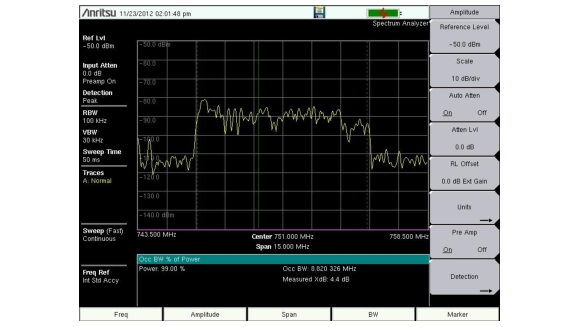MA2700A InterferenceHunter Help : Spectrum Analyzer : Occupied Bandwidth Measurement

Occupied Bandwidth Measurement
Occupied Bandwidth (OBW) is a common measurement performed on radio transmitters. This measurement calculates the bandwidth containing the total integrated power occupied in a given signal bandwidth. There are two different methods of calculation depending upon the technique used to modulate the carrier.
% Integrated Power Method: The occupied frequency bandwidth is calculated as the bandwidth containing the specified percentage of the transmitted power.
> dBc Method: The occupied frequency bandwidth is defined as the bandwidth between the upper and lower frequency points at which the signal level is a desired number of dB below the peak carrier level.
Required Equipment
Test Port Extension Cable, Anritsu part number 15NNF50‑1.5C
30 dB, 50 Watt, bi‑directional, DC –18 GHz, N(m) – N(f), Attenuator, Anritsu 42N50A‑30 (required if the power level being measured is > + 30 dBm)
Procedure
1. Using the test port extension cable and the 30 dB, 50 watt, bi‑directional attenuator (if needed) connect the RF In port to the appropriate transmitter test port or signal source.
2. Press the Freq main menu key followed by the Center Freq submenu key and enter the center frequency using the keypad, the arrow keys, or the rotary knob. If entering a frequency using the keypad, the submenu key labels change to GHz, MHz, kHz, and Hz. Press the appropriate units key. Pressing the Enter key has the same effect as the MHz submenu key.
3. If an attenuator was connected in Step 1, then press the Amplitude main menu key then press the RL Offset submenu key, enter 30 then select dB External Loss to compensate for the loss in the attenuator.
4. Press the Amplitude main menu key then press the Reference Level submenu key to set the appropriate reference level.
5. Press the Atten Lvl submenu key to set the input attenuation level or leave Auto Atten set to On.
6. Press the BW main menu key to set the resolution bandwidth and video bandwidth if desired.
7. Press the Shift key then the Measure (4) key. Then press the Power and Bandwidth submenu key, and press the OCC BW submenu key. Choose the measurement method (% Int Pwr or > dBc) by pressing the Method submenu key. The selected method is underlined.
8. Press the dBc or % submenu keys to adjust the settings as needed. Common values are 99% and 30 dBc.
9. Press the On/Off submenu key to start the measurement. An information box appears below the graph while occupied bandwidth measurement is on.
Figure: Occupied Bandwidth Results Using the % of Power Method shows the occupied bandwidth results using the percent of power method on a WCDMA signal. Occupied Bandwidth is a constant measurement; after it is turned on, it remains on until it is turned off by pressing the On/Off submenu key again. Occupied bandwidth is calculated at the end of each sweep.